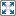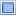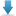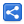# Python Basic Syntax

[Last Updated: Feb 1, 2022]

Python is designed to be highly readable.

## Indentation

Most of the programming languages like C, and Java use curly brackets { } to define a block of code. Python uses indentation to define block of code.

Python is an indentation style language, that is it uses indentation of blocks of code to convey program structure.

In Python, a colon is required at the beginning of every block of code.

### Example

Following example shows how we use indentation with a if/else block:

#### scripts/python-indentation-example.py

```x = 3
if x < 5:
print("In if block")
print("Number less than 5")
else:
print("In else block")
print("Number more than 5 inclusively")
```

#### Output

`In if blockNumber less than 5`

If our indentation is not good then:

#### scripts/python-indentation-error.py

```x = 3
if x < 5:
print("Number less than 5")
else:
print("Number more than 5 inclusively")
```

#### Output

`  File "d:\example-projects\python\python-basic-syntax\scripts\python-indentation-error.py", line 3    print("Number less than 5")    ^IndentationError: expected an indented block after 'if' statement on line 2`

## No semicolons

As seen in above example, Python does not require to use ; at each statements.

We can use semicolons to use multiple statements in the same line:

#### scripts/python-semi-colons.py

```x = 3; y = 5
print(x); print(y); print(x + y)
```

`358`

# Example Project

Dependencies and Technologies Used:

• Python 3.10.2
 Python Basic SyntaxSelect AllDownload• python-basic-syntax
• scripts
• python-indentation-example.py

 Share